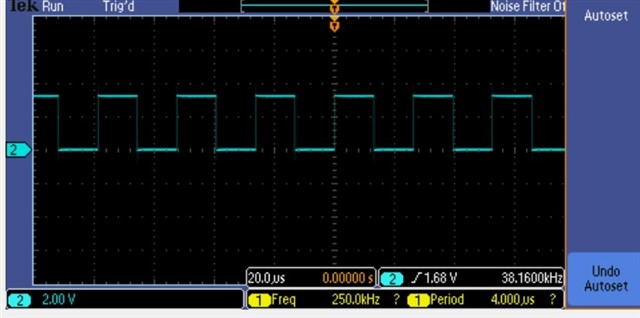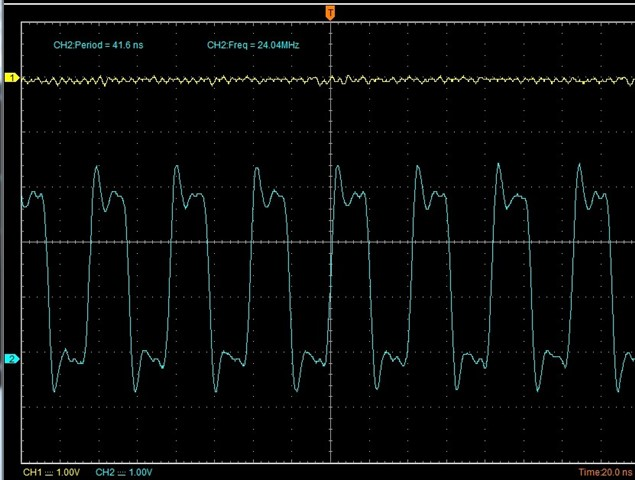If you have a related question, please click the "Ask a related question" button in the top right corner. The newly created question will be automatically linked to this question.

# TLV320AIC3212: WCLK is different from setting

Part Number: TLV320AIC3212

Dear experts,

We want to set WCLK at 44.1KHz with MCLK=24MHz.

However, after we set the parameters, the WCLK output was not correct ( it shows in 38kHz).

The parameters are set as follows: NDAC=8, MDAC=1, DOSR=128, J=4, R=4, P=5, D=704, PLL_CLKIN_DIV=2 (PLL CLK selects MCLK)

Based on formula DAC_FS=DAC_CLKIN/(NDAC*MDAC*DOSR)

DAC_CLKIN=PLL_CLK_out=(PLL_CLKIN*R*J.R)/(P*PLL_CLK_in_DIV)=(24000000*4*4.704)/(5*2)=45158400

DAC_FS=45158400/(8*1*128)=44100

The waveform is as below:

WCLK: (38KHz)MCLK: (24MHz)Is there anything we miss or something wrong in our calculation so that we could not get 44.1KHz WCLK output?

Thank you.

Best Regards,

Cindy